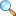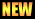# Unit 02: Theory of Quadratic Equations

On this page the solutions and things related to Unit 02: Theory of Quadratic Equations of Mathematics 10 (Science Group) (Matric) are given. These notes are send by Mr. Amir Shehzad, we are very thankful to him for sending these notes.

In this unit students will learn how to

• define discriminant $(b^2-4ac)$ of quadratic expression $ax^2+bx+c$.
• find discriminant of a given quadratic equation.
• discuss the nature of roots of a quadratic equation through discriminant and verify the results by solving the equation.
• determine the nature of the roots of a given quadratic equation and verify the result by solving the equation.
• Exercise 2.8 |View Online | Download PDF• matric/10th_science/unit02